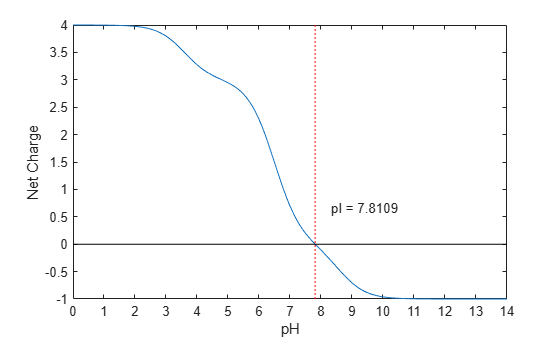# isoelectric

Estimate isoelectric point for amino acid sequence

## Syntax

```pI = isoelectric(SeqAA) [pI Charge] = isoelectric(SeqAA) isoelectric(..., 'PropertyName', PropertyValue,...) isoelectric(..., 'PKVals', PKValsValue) isoelectric(..., 'Charge', ChargeValue) isoelectric(..., 'Chart', ChartValue) ```

## Arguments

 `SeqAA` Amino acid sequence. Enter a character vector, string, or a vector of integers from the table Mapping Amino Acid Letter Codes to Integers. Examples: `'ARN'` or `[1 2 3]`. `PKValsValue` Character vector or string specifying a file name or path and file name of a PK file containing a table of pK values for amino acids, which .`isoelectric` uses to estimate the isoelectric point (`pI`) of an amino acid sequence. For an example of a PK file format, type `edit Emboss.pK` in the MATLAB® command line. `ChargeValue` Property to select a specific pH for estimating charge. Enter a number between `0` and `14`. Default is `7.2`. `ChartValue` Controls the plotting a graph of charge versus pH. Enter `true` or `false`.

## Description

`pI = isoelectric(SeqAA)` returns the estimated isoelectric point (`pI`) for an amino acid sequence using the following pK values:

```N_term 8.6 K 10.8 R 12.5 H 6.5 D 3.9 E 4.1 C 8.5 Y 10.1 C_term 3.6```

The isoelectric point is the pH at which the protein has a net charge of zero.

`[pI Charge] = isoelectric(SeqAA)` returns the estimated isoelectric point (`pI`) for an amino acid sequence and the estimated charge for a given pH (default is typical intracellular pH `7.2`).

The estimates are skewed by the underlying assumptions that all amino acids are fully exposed to the solvent, that neighboring peptides have no influence on the pK of any given amino acid, and that the constitutive amino acids, as well as the N- and C-termini, are unmodified. Cysteine residues participating in disulfide bridges also affect the true pI and are not considered here. By default, `isoelectric` uses the EMBOSS amino acid pK table, or you can substitute other values using the property `PKVals`.

• If the sequence contains ambiguous amino acid characters (b z * –), `isoelectric` ignores the characters and displays a warning message.

```Warning: Symbols other than the standard 20 amino acids appear in the sequence.```
• If the sequence contains undefined amino acid characters (`i j o`) , `isoelectric` ignores the characters and displays a warning message.

```Warning: Sequence contains unknown characters. These will be ignored.```

``` isoelectric(..., 'PropertyName', PropertyValue,...)``` defines optional properties using property name/value pairs.

`isoelectric(..., 'PKVals', PKValsValue)` uses pK values stored in a `PKValValues`, a PK file, to estimate the isoelectric point (`pI`) of an amino acid sequence. For an example of a PK file format, type ```edit Emboss.pK``` in the MATLAB command line.

`isoelectric(..., 'Charge', ChargeValue)` returns the estimated charge of a sequence for a given pH (`ChargeValue`).

`isoelectric(..., 'Chart', ChartValue)` when `ChartValue` is `true`, returns a graph plotting the charge of the protein versus the pH of the solvent.

## Examples

collapse all

Calculate the isoelectric point using the pK values stored in a PK file and plot the charge against the pH for a short sequence.

`isoelectric('PQGGGGWGQPHGGGWGQPHGGGGWGQGGSHSQG','PKVal','Emboss.pK','CHART',true)````ans = 7.8109 ```

Also estimate its charge at a specific pH.

`[pI,Charge] = isoelectric('PQGGGGWGQPHGGGWGQPHGGGGWGQGGSHSQG','Charge',7.3)`
```pI = 7.8109 ```
```Charge = 0.3629 ```

## Version History

Introduced before R2006a